# Entropy change of a hot, falling object

## Homework Statement

A shipyard worker drops a hot steel rivet (mass 125g, temperature 350 degrees C)
into a river at temperature 5 degrees C, a distance 30m below. Stating any assumptions
you make, calculate the entropy change of the universe as a result of this event.
(specific heat capacity of steel ~0.4 J g-1 K-1).

## Homework Equations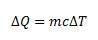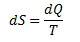## The Attempt at a Solution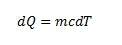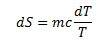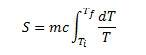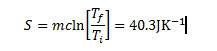If anybody could tell me if I'm on the right lines at all or what the heck to do with the fact that the rivet's being dropped from 30m I'd greatly appreciate it. Thanks!

Last edited:

## Answers and Replies

dynamicsolo
Homework Helper
You have traced the change in entropy of the rivet, but I believe there are two more things you need to look at in the "universe" (essentially the rivet-Earth system). How much heat has been added to the river by the change in gravitational potential energy in the rivet-Earth system? Also, the river absorbs the heat of the rivet with virtually no temperature change (it's large enough to serve as a "heat reservoir"), so what is the change in the entropy of the river from that?

So there is the entropy change of the cooling rivet plus the entropy change from the change in mechanical energy plus the entropy change from the heat transfer from rivet to river.

Thanks for the reply.

So, as I see it, so far I've worked out the decrease in the entropy of the rivet.

The heat flowing into the reservoir from the rivet cooling will be the same as the heat loss from the rivet:That gives me 17250 J.

Then, if we assume the temperature of the reservoir doesn't change, then, using the temperature of the river in Kelvins for T: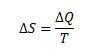That gives me an increase of entropy in the river as 62.1 JK-1

Finally, the change in gravitational potential energy is: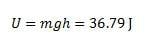If we assume that this is all converted to heat in the river we can work out a second component for the increase in entropy. I get S = 0.13 JK-1.

Overall this gives me a net increase in the entropy of the universe as S = 21.93 JK-1.

Is this the right track or have I completely messed up?

dynamicsolo
Homework Helper
I agree with those results to the first decimal place (since I rounded off only at the end), so we get a net increase in entropy for the "universe" of 21.9 J/K .

It is a reasonable safe assumption that just about all of the potential energy change is transferred into heating the river. The "splash" at impact displaces water and ejects some of it upward (briefly), as well as producing (a minute amount of) acoustical energy. But since practically all the water falls back into the river, that mechanical energy winds up heating the river after all. (We can extend "the universe" to include the air over the river, but the tiny amounts of water scattered as microdroplets and vapor and of sound energy probably contribute additions to entropy scarcely worth pursuing...)